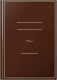Books Books 1 - 9 of about 9 editions of Introduction to Mathematical Statistics.## Introduction to Mathematical Statistics

Robert V. Hogg, Allen Thornton Craig - Mathematical statistics - 2003 - 564 pages## Introduction to Mathematical Statistics

Robert V. Hogg, Allen Thornton Craig - Mathematical statistics - 2003 - 564 pages## Introduction to Mathematical Statistics

Robert Vincent Hogg, Allen Thornton Craig - Mathematical statistics - 1998 - 564 pages## Introduction to Mathematical Statistics

Mathematical statistics - 1995 - 575 pages## Introduction to Mathematical Statistics

Robert V. Hogg, Allen T. Craig - Mathematical statistics - 1995 - 564 pages## Introduction to Mathematical Statistics

Robert V. Hogg, Allen T. Craig - Probability & statistics - 1995 - 564 pages## Introduction to Mathematical Statistics

Robert V. Hogg - Mathematical statistics. - 1995 - 564 pages
An exceptionally clear and impeccably accurate presentation of statistical applications and more advanced theory. Included is a chapter on the distribution of functions of ...## Introduction to Mathematical Statistics

Robert V. Hogg - Mathematical statistics. - 1995 - 564 pages
An exceptionally clear and impeccably accurate presentation of statistical applications and more advanced theory. Included is a chapter on the distribution of functions of ...